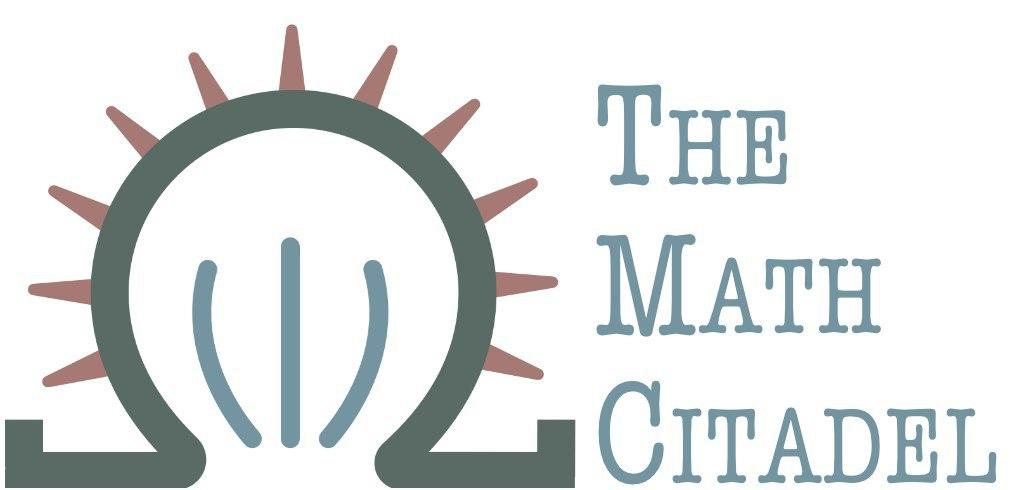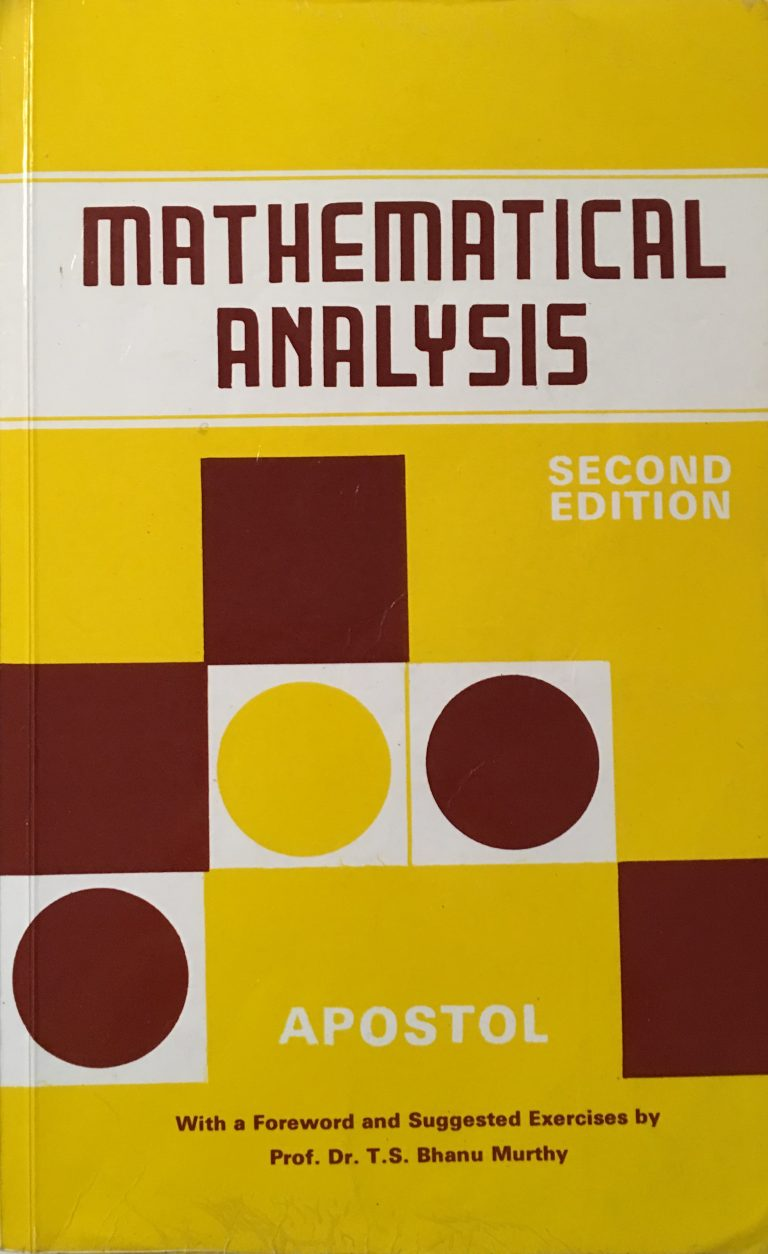## Book Review: Mathematical Analysis, 2nd edition

### Author: T. Apostol

#### Reviewed by: R. Traylor## Ranking

All ranking is done on a scale of 1-5, with 5 as the best.
Attribute Rank
Difficulty 3
Proof Quality 5
Self-Study 4
Good for Teaching? 5
Quality of Exercises 5

## Topics Covered

• Real and complex number systems
• Basic Set Theory
• Point-Set Topology -
• Open and closed sets
• Bolzano-Weierstrass Theorem
• Cantor Intersection Theorem
• Lindelof Covering Theorem
• Compactness and metric spaces
• Limits and Continuity
• Derivatives
• Bounded Variation and Total Variation
• Riemann-Stieltjes Integration
• Residue Calculus
• Infinite Series and Products
• Convergence and tests for convergence
• double sequences and double series
• Cesaro summability
• Sequences of Functions
• types of convergence
• power series
• Lebesgue Integration
• Levi monotone convergence theorems
• Fourier series
• Multivariable Differentiable Calculus
• Implicit Functions and Extrema
• Multiple Integration: Riemann and Lebesgue